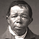6111 views
Hello everyone,

today I'd like to share a script, which enables you to use external logic to plot levels on your chart.

How does it work?

The concept is based on two scripts. One script, which uses an external input as a trigger to print a new level and one script that calculates an output, which will be fetched.

Sounds complicated? It really is not! Let's take a closer look.

```// This source code is subject to the terms of the Mozilla Public License 2.0 at https://mozilla.org/MPL/2.0/

//@version=4
study("RSI OS/OB")
l = input(14, "RSI Length")
ob = input(70, "Overbought")
os = input(30, "Oversold")

r = [__tag__=relativestrengthindex]rsi[/__tag__](close, l)

hline(ob)
hline(os)
plot(r, "RSI", color=color.orange)

// The following plot produces an output, which will be fetched the "External Level"-script.
// It evaluates to one of the following three values: 1.0, -1.0 or 0.0
plot(crossover(r, ob) ? 1.0 : crossunder(r, os) ? -1.0 : 0.0, "Output", transp=100)```

The example script above uses an RSI and two threshold levels (70 and 30). The logic here is, that whenever the RSI is crossing down the lower threshold or crossing up the upper threshold we'd consider the current movement to be either oversold or overbought. Therefore, it's a point of interest, which we could visualize with a level.
The script creates an output when the crossover or crossunder of a threshold happens. A crossover would result in a value of 1.0, a crossunder in a value of -1.0. In all other cases the value would be 0.0.

The output of the RSI script would then be used as an input of the External Level script, which has a "Source"-parameter in its input-section. If the fetched input shows 1.0, then the script prints a resistance level . If it shows -1.0 a support level will be printed. And that's basically it. A very simple approach to print levels on your chart with an infinite number of use cases.
For example, you could use fetch outputs from a MACD script, MA script, outputs based on volume or price movement. Just remember the output has to evaluate to either 1.0 or -1.0 and has to be selected in the input-section.

Hope that might be useful to some of you :)

Please click the "Like"-button and follow me for future open-source script publications.

If you are looking for help with your custom PineScript development, don't hesitate to contact me directly here on Tradingview or through the link in my signature :)
http://www.pascal-simon.de - Custom indicator development...come on ... I'm the only one with that error message from the Source part? "

Line 10 Syntax Error //r = rsi(close, l)Prospector136
@Prospector136, "r = rsi(close, l) " this line shouldn't cause any error. In the above snippet, it's a formatting error, caused due to posting code in the description. What you posted here in the comments, looks like my own code, which compiles just fine.